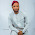Tuesday, 28 March 2017

Updated Method In Calculating the Number Of Roofing Sheets Needed For Your Building

As a client, it is only right that you have some idea on the quantity of materials that would be required for the completion of your building or structure. It is based on this that i have built this blog and today, i am going to make it easy for you to calculate the number of roofing sheets required for your building project.

So How do you calculate the number of Aluminum roofing sheets, Long span roofing sheets required for your building?

Lets begin this way...

You understand that around us, the pitch roof is the most common type of roof for everyone. A pitched roof has its appex divided.

I decided to use the pitched roof simply because it is one of the most easiest to analyse. In order to be able to calculate the number of roofing sheets required for your building, you'll need to ask your contractor or engineer the actual size of his intended roofing sheet. This is necessary especially if you're sure your roof is not going to be Long span aluminum sheets which are long enough to span through the the entire length of the roof

So, Lets begin by measuring the length of the building and call it say alphabet ‘x’, measure the breadth and call it alphabet ‘y’.

To get an approximate surface area we will add an arbitrary value for the difference in length due to the pitch or depth of roof. So lets say for a house of total length of the front is 1.8m or 60ft and side which is the longer part is 27m or 90ft.

Area =length of front of house x side of house=18 x 27=486m2

Add allowance for the pitch or depth 20% of Area=20% + 486m =583.2m2

Now find out the length and breadth of each roofing sheet and also multiply length by breadth.

Assuming the ceilling sheet is 3.6m in length and 1.8m in breadth

Then area of roofing sheet =3.6 x 1.8 =6.48m2

Therefore total numbers of roofing sheets needed approximately is

(Area of building + Allowance)/area of one roofing sheet =583.2/6.48m

=90 sheets

1.2.3.